l

2017年2月22日 星期三

BDD（7）：使用Transform讓稅金同時支援5%和0.05表達方式

Feb. 21 22:00~00:00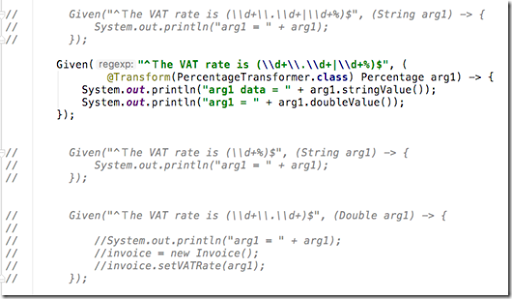▲好亂的程式碼XD

Given The VAT rate is 0.05

Given("^Ｔhe VAT rate is (\\d+\\.\\d+)\$", (Double arg1) –> {

Given The VAT rate is 5%

Given("^Ｔhe VAT rate is (\\d+)%\$", (Integer arg1) –> {

Given("^Ｔhe VAT rate is (\\d+%)\$", (String arg1) –> {

***

▼以下scenario例子故意重複撰寫兩次「The VAT rate is」，第一次使用0.05，第二次使用5%。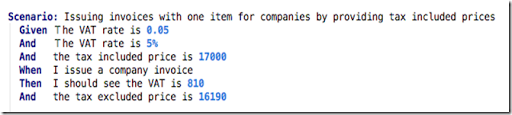▼這個step definition可以同時對應到以上兩種情況。

Given("^Ｔhe VAT rate is (\\d+\\.\\d+|\\d+%)\$", (String arg1) -> {
System.out.println("arg1 = " + arg1);
});

▼輸出結果為：

arg1 = 0.05
arg1 = 5%

invoice.setVATRate(arg1);

***

▼Cucumber的Transform功能可以協助解決這個問題，首先我們新增Percentage（百分比）類別，它可以接受0.05或5%這種格式，然後轉成double型態。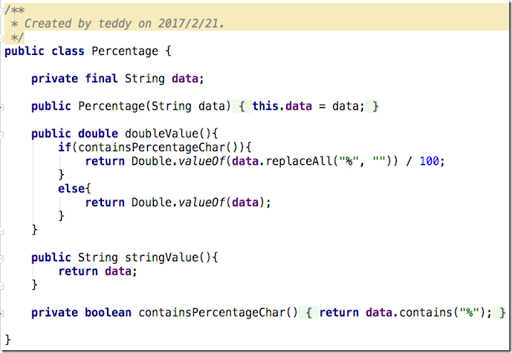▼接著新增PercentageTransformer類別，它必須繼承自Cucumber的Transformer類別，然後覆寫transform method，以便把一個字串轉成Percentage類別。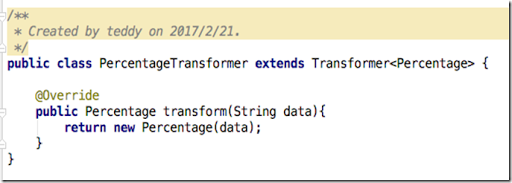▼到這裡準備工作都已就緒，最後只要把step definition改一下，從原本傳入參數接受String型別改成接受Percentage型別，然後在它前面加上@Transform(PercentageTransformer.class)告訴Cucumber如何把step裡面的字串（也就是0.05或5%）透過PercentageTransformer類別轉成Percentage型別傳入。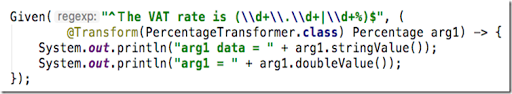▼輸出結果為：

arg1 data = 0.05
arg1 = 0.05
arg1 data = 5%
arg1 = 0.05

***